# Linear momentum

## Definition

### For a single body in pure translation

The linear momentum of a body is the vector$m\overline{v}$, where$m$ is the mass of the body and$\overline{v}$ is the velocity of the body.

### For a system of bodies, each in pure translation

The linear momentum of a system is the sum of the linear momenta of all the bodies in that system. If the system comprises$n$ bodies with masses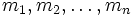$m_1, m_2, \dots, m_n$ respectively and velocities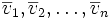$\overline{v}_1, \overline{v}_2, \dots, \overline{v}_n$ respectively, the total linear momentum is given by: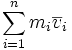$\! \sum_{i=1}^n m_i\overline{v}_i$

This is a vector summation.

### For a single body undergoing motion that is not purely translation

In this case, we integrate the velocity vector over a mass differential: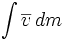$\int \overline{v} \, dm$

This is equivalent to integrating the product of velocity and density over a volume differential: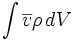$\int \overline{v} \rho \, dV$

## Units and dimensions

MLT dimensions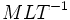$MLT^{-1}$: MLT;1;1;-1 (same as those of impulse)
SI units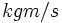$kgm/s$ (kilograms meter per second) or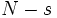$N-s$ (Newton-second)